International
Tables for
Crystallography
Volume D
Physical properties of crystals
Edited by A. Authier

International Tables for Crystallography (2006). Vol. D, ch. 1.1, p. 4

## Section 1.1.1.3. The matrix of physical properties

A. Authiera*

aInstitut de Minéralogie et de la Physique des Milieux Condensés, Bâtiment 7, 140 rue de Lourmel, 75015 Paris, France
Correspondence e-mail: aauthier@wanadoo.fr

#### 1.1.1.3. The matrix of physical properties

| top | pdf |

Each extensive parameter is in principle a function of all the intensive parameters. For a variation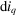of a particular intensive parameter, there will be a variation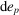of every extensive parameter. One may therefore write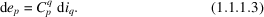The summation is over all the intensive parameters that have varied.

One may use a matrix notation to write the equations relating the variations of each extensive parameter to the variations of all the intensive parameters: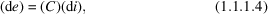where the intensive and extensive parameters are arranged in column matrices, (di) and (de), respectively. In a similar way, one could write the relations between intensive and extensive parameters as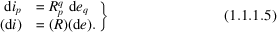Matrices (C) and (R) are inverse matrices. Their leading diagonal terms relate an extensive parameter and the associated intensive parameter (their product has the dimensions of energy), e.g. the elastic constants, the dielectric constant, the specific heat etc. The corresponding physical properties are called principal properties. If one only of the intensive parameters,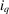, varies, a variationof this parameter is the cause of which the effect is a variation,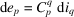(without summation), of each of the extensive parameters. The matrix coefficients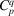may therefore be considered as partial differentials: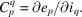The parametersthat relate causesand effectsrepresent physical properties and matrix (C) is called the matrix of physical properties. Let us consider the following intensive parameters: T stress, E electric field, H magnetic field, Θ temperature and the associated extensive parameters: S strain, P electric polarization, B magnetic induction, σ entropy, respectively. Matrix equation (1.1.1.4)may then be written: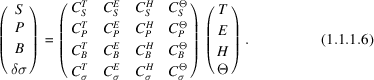The various intensive and extensive parameters are represented by scalars, vectors or tensors of higher rank, and each has several components. The terms of matrix (C) are therefore actually submatrices containing all the coefficientsrelating all the components of a given extensive parameter to the components of an intensive parameter. The leading diagonal terms,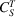,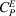,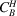,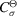, correspond to the principal physical properties, which are elasticity, dielectric susceptibility, magnetic susceptibility and specific heat, respectively. The non-diagonal terms are also associated with physical properties, but they relate intensive and extensive parameters whose products do not have the dimension of energy. They may be coupled in pairs symmetrically with respect to the main diagonal: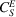and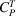represent the piezoelectric effect and the converse piezoelectric effect, respectively;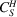and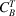the piezomagnetic effect and the converse piezomagnetic effect;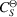and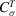thermal expansion and the piezocalorific effect;and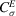the pyroelectric and the electrocalorific effects;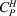and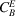the magnetoelectric effect and the converse magnetoelectric effect;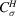and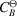the pyromagnetic effect and the magnetocalorific effect.

It is important to note that equation (1.1.1.6)is of a thermodynamic nature and simply provides a general framework. It indicates the possibility for a given physical property to exist, but in no way states that a given material will exhibit it. Curie laws, which will be described in Section 1.1.4.2, show for instance that certain properties such as pyroelectricity or piezoelectricity may only appear in crystals that belong to certain point groups.